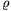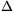Mathematical and Physical Journal
for High Schools
Issued by the MATFUND Foundation
 Already signed up? New to KöMaL?

#Problem P. 3819. (September 2005)

P. 3819. The density of a uniform rod with cross-section A is, its specific heat capacity is c and the coefficient of its linear expansion is. Calculate the amount of heat that should be added in order to increase the length of the rod byl.

(3 pont)

Deadline expired on October 11, 2005.

### Statistics:

 271 students sent a solution. 3 points: 226 students. 2 points: 23 students. 1 point: 9 students. 0 point: 4 students. Unfair, not evaluated: 9 solutionss.

Problems in Physics of KöMaL, September 2005